# Scala Tutorial

Rating: 4.6

3476
1. Share:
Apache Scala Articles

Scala is a contemporary paradigm programming language developed for expressing general programming patterns in an elegant, type-safe and concise way. Scala easily incorporates functional languages and object-oriented features, so it has all the features that are present in the functional and object-oriented programming languages like C, Java, Python, etc. This Scala tutorial will help you in learning the basic concepts of Scala Programming.

Want to become a Scala Certified Professional? Visit here to learn Scala Training

Scala Tutorial for Beginners - Updated 2020

In this tutorial, we will discuss the following topics:

## What is Scala?

Scala is an object-oriented programming language that is fast, efficient and compact. We compile the Scala Programs by using the scala compiler.  In Scala, everything is function and object. Scala is useful for handling the huge-amount of Big data.

### Scala Features

Following are the important features of Scala:

#### #1) Type Inference

In Scala, we don't need to specify the data type and return type of the functions. We can determine the return type of the function through the last expression type that is available in the function.

#### #2) Singleton Object

In Scala, we will not have any static methods or variables. Scala utilizes Singleton Object, which is basically a class with just one object in the source code. By using the “object” keyword, we can declare the Singleton Object.

#### #3) Lazy Computation

Scala assesses the expression when they are necessary. By using the "Lazy" keyword, we can declare the lazy variables. The lazy computation enhances the performance.

#### #4) Immutability

In Scala, every variable we declare is immutable. Immutable means we cannot change the value. Immutable variables assist in handling the concurrency control that needs managed data.

 Related Article: Easy to Learn Programming Languages ​in Data Science

#### #5) Concurrency Control

Scala gives the regular library that contains the actor model. By using the actor, we can develop the concurrency code. To deal with the concurrency, Scala has a separate platform called "Akka".

#### #6) High Order Functions

High Order Functions take a function as an argument and returns a function. A function that works with another function is known as High Order Function.

#### #7) String Interpolation

From Scala 2.10.0, Scala provides a new method for creating the strings from our data, and that method is known as String Interpolation. The string interpolation enables the users to include variable references in the processed string literals.### Basic Syntax of Scala Programming

If you have good knowledge of Java, then it is easy for you to learn the Scala programming language. We consider a scala program as a collection of objects that interact by calling each other's methods. Following are the components of a scala program:

Class: A class is a blueprint or a template that explains the states or behaviours that are associated with that class.

Object: Objects contain behaviours and states. Object is an instance of the class.

Method: Generally, the method is behaviour. A class can have many methods. In methods, we can develop logics and manipulate the data.

Closure: Closure is a function, and its return value depends on the values of the variables that we declare outside this function.

Field: Every object will have a distinct group of instance variables, and those variables are known as fields.

Traits: A trait binds the field and method definitions, and we can reuse them by blending them into the classes.

Read these latest Scala interview questions that helps you grab high-paying jobs!

### How to write a Scala Program

For writing a scala program in our machine, we need to perform the following steps:

Step1: Check Java Installation

For checking the java installation in our machine, we need to run any one of the following commands in the command console. If we have Java in our machine, then the following commands will display the current specifications and version of our java installation.

• If our machine has windows operating system, then we have to execute the following command:

> java -version

• If our machines have the Linux Operating system, then we have to execute the following command:

\$java -version

If we don't have Java in our system, we can download and install it.

Step2: Setting Java Environment

After installing Java, we have to set its environment.

For setting the java environment, we have to configure the "JAVA_HOME" environment variable for targeting the location of the directory where we installed the Java.

After installing and configuring Java, we have to download the Scala. After downloading the Scala, we can install it by executing the below command in the command console:

>java -jar -scala2.11.5- installer.jar>

After installing the Scala, we can see the scala version by executing the following command in the command console:

> Scala -version

After downloading and installing the Scala, we can write and execute the Scala Programs.

Sample Scala Program:

``````Object Scala1{
Def main(args:Array[String]){
println" Hai".
}
}
``````

Note: In Scala Programming, “;” end line character is optional.

We save the above program with "Scala1.scala" name.

After saving the program, we will compile the code in the following way:

scalac Scala1.scala

After compiling, we execute the program:

scala Scala1

When we execute the program, we get the following output:

Hai

### Data Types in Scala

Scala has all the data types that are available in Java. Following are the data types available in the Scala:

Byte, Int, Short, Float, Long, Char, Double, String, Boolean.

#### 1) Variables

In any Programming language, variables act as memory locations for storing the values. When we create a variable, we have to hold some space in memory.

According to the variable data type, the compiler assigns the memory and determines what we can store in the memory. In Scala, we have a syntax to declare the variables. We can specify the variables as a variable or a constant.

#### 2) Variable Declaration

In the following example, we declare "myVar1" variable through the "var" keyword. The "myVar1" variable can change the value, so it is known as a mutable variable.

Example1:

var myVar1 : String = “Food”

In the below example, we declare "myVar2" variable through the "val" keyword. The "myVar2" variable cannot modify the value, so it  is known as an immutable variable.

Example2:

Val myVar2 : String = “Food”

#### 3) Variable Scope

In Scale, variables have three types of scopes, according to the place where we use them. The variables can be method parameters, local variables and fields.

a) Fields

Fields are the variables that are members of an Object. We can access the fields from the methods that are available in the object. We can access the fields from outside the object depending on the access modifiers of the variables.

b) Method Parameters

We use Method Parameters for sending the values inside a method when we invoke a method. We can access the method parameters only from inside the method.

c) Local Variables

We can declare the local variables inside the method. They are accessible only from inside the method.

### Scala OOPS Concepts

#### 1) Object

Object is a real-time entity. It includes behaviour and state. Objects have the following features:

Behaviour: The functionality that an object carries out is called its behaviour.

State: States are the data values of Objects.

#### 2) Class

Class is a blueprint or template, and it is called as a group of Objects of the same type.

A Class contains the following things:

• Member Method
• Data Member
• Block
• Constructor
• Nested Class

#### 3) Inheritance

Scala has different kinds of inheritances, that share plenty of features present in Java. We can inherit from both traits and classes. By using the "extends" keyword, we can inherit the members of one class to another class. We can inherit the data members from multiple classes also.

Output:

Syntax of Inheritance is as follows: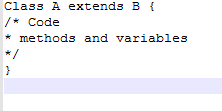Example for Inheritance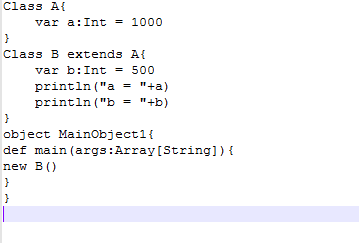Output: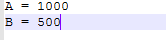#### 4) Scala Constructor

Scala has auxiliary and primary constructors.

a) Scala Primary Constructor

In Scala, if we don’t define any constructor, the compiler automatically produces a constructor that is called as primary constructor. The statements present in a class are the components of a constructor. The primary constructor is also called as default constructor.

Example: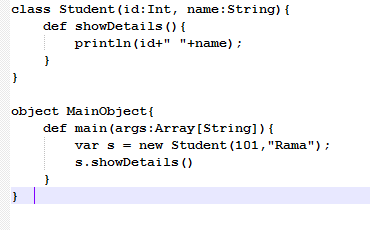Output: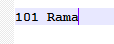b) Scala Auxiliary(Secondary) Constructor

In Scala, we can create an unlimited number of auxiliary constructors in the same class. From the auxiliary constructor, we can invoke the primary constructor. By using the “this” keyword, we can call a constructor from another constructor.

Method Overloading enables us to declare methods with similar names but has different data types or parameters. It assists us in optimizing the code.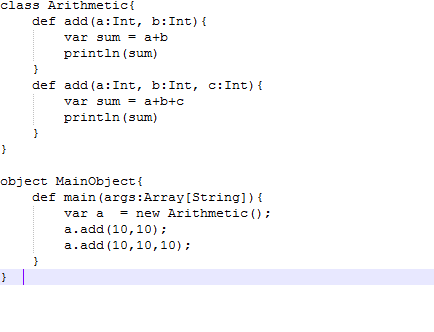Output: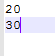#### 6)  “this” keyword

In Scala, we use “this” keyword for referring to the existing objects. We use “this” keyword for invoking instance methods, variables, and constructors.

Example:

``````class This{

var a:Int = 0

var emp: String = “”

def this(a:Int, emp:String){

this()

this.a = a

this.emp = emp

}

def print(){

println(a+ “ “ +emp)

}
}

object Main1{

def main(args: Array[String]) {

var c = new This{100, “Vijay”)

c.print()

}
}
``````

Output:

100 Vijay

#### 7) Method Overriding in Scala

When the subclass contains a method with the same name as specified in the parent class, then it is called as Method Overriding. When the subclass has to give implementation to the method that is declared in the parent class, then it overrides the method from the parent class.

For overriding the methods from the parent class, we should use override annotation or “override” keyword.

Example:

``````class Bike{

def work(){

println(“Bike is working”)

}
}

Class Bike extends Car{.

override def work(){

println(“Car is working”)

}
}

object Main1{

def main(args: Array[String]){

var c = new Car()

c.work()

}
}
``````

Output:

Car is Working

Multithreading is a mechanism that runs multiple threads concurrently. It enables us to execute multiple tasks independently. We can accomplish multitasking through multithreading. Thread is a light-weight subprocess that holds less space(memory).

In Scala, we use multithreading for developing concurrent applications. The Scala Multithreading is similar to Java multithreading except for Scala language syntax. We create threads by extending the Thread class or by implementing the Runnable Interface.

In the following screenshot, we can see a sample program of Thread Concept: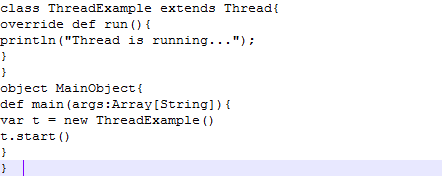Out put: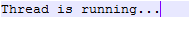It is the period in which the thread begins and ends. The thread life cycle has different phases or states like New, Runnable, Block, Terminate, etc. Thread class contains different methods to control the thread states.

#### 9) “Final” Keyword

We use the “Final” keyword for preventing the inheritance of base class members into the derived class. We can define final methods, classes and variables also.

Sample Program for “Final” keyword: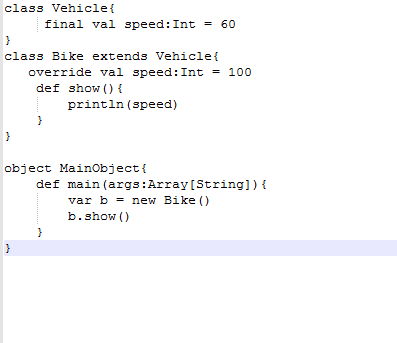### Operators

Operators are symbols that tell the compiler to carry out particular logical or arithmetic operations. Scala Provides the following operators:

#### 1) Arithmetic Operators

Scala supports the following Arithmetic Operators. For instance, let us consider Variable “C” stores 40 and Variable “D” stores 20, then

 Operator Explanation Example - We use the “-” operator for subtracting the second operand from the first operand. C-D gives 20. + We use the “+” operator for adding two operands. C+D gives 60. * We use “*” operator for multiplying two operands C*D gives 800. / “/” operator divides the numerator by denominator. C/D gives 2. % “%”  operator determines the remainder after dividing a number with another number C%D gives 0.

#### 2) Relational Operators

Scala Language supports the following relational operators. For instance, let us consider variable “C” stores 30 and variable “D” stores 40. Then

 Operator Explanation Example == The “==” operator checks whether the given two operands are equal or not. If they are equal, then the result is true, else the result is false. (C==D) is not true. != The “!=” operator checks whether the given two operands are equal or not. If they are not equal, then the result is true, else the result is false. (C!=D) is true. < The “< “ operator checks whether the value of the left operand is less than the value of the right operand or not. If yes then the result is true, else, it is false. (C The “>” operator checks whether the value of the left operand is greater than the value of the right operand or not. If yes then the result is true, else, it is false. (C>D) is false. <= The “<=” operator checks whether the value of the left operand is less than or equal to value of the right operand. If yes, then the result is true, else, it is false. (C<=D) is true >= The “>=” operator checks whether the value of the left operand is greater than or equal to the value of the right operand. If yes, then the result is true, else it is false. (C>=D) is false.

#### 3) Logical Operators

Scala Program Language supports the following logical operators. For Instance, let us consider variable “C” stores 1, and variable “D” stores 0, then:

 Operator Explanation Example && The “&&” operator is known as the Logical AND operator. If the given two operands are non-zero, then the result becomes true. (C&&D) is false || The “||” operator is known as the Logical OR operator. If one of the two operands is non-zero, then the result becomes true. (C || D) is true ! The “!” operator is known as the Logical NOT operator. NOT operator invertes the logical state of the operand. If the condition is false, then the NOT Operator will make it true. !D is true.

#### 4) Bitwise Operators

The bitwise operators operate on the bits and carry out bit by bit operations. The truth tables for different bitwise operators are as follows:

 R S R&S R|S R^S 0 0 0 0 0 0 1 0 1 1 1 1 1 1 0 1 0 0 1 1

R&S- Bitwise AND

R|S- Bitwise OR

R^S- Bitwise NOT

#### 5) Assignment Operators

Scala Programming Language supports the following assignment operators:

=, +=, -=, /=, *=, <<=, >>=, &=, |=.

Scala Operators Precedence

Operators Precedence decides the arrangement of the terms in the expression. The operator precedence impacts how we evaluate the expression. Some operators will have higher precedence than the others. For example, the division operator will have higher precedence than the multiplication operator.

For Instance, a = 20 / 10 * 3, here, we assign 6 to a, because “/” division operator has higher precedence than the “*” multiplication operator.

### Scala Conditional Statements

Following are the conditional statements of Scala Programming Language

#### 1) “If” Statement

“If” statement contains a boolean expression and one or more statements.

Syntax of “If” is as follows:

``````if(boolean_expression) {

Statements;

}
``````

If the boolean expression is true, then the code present in the “If” block gets executed. If the boolean expression is not true, then the code present at the end of the “If” block gets executed.

Example:

``````object If{

def main(args: Array[String]) {

var x=30;

if(x>20) {

printf(“If Statement Example”);

}
}
}
``````

As the boolean expression or condition is true, we will get the following Output:

If Statement Example.

#### 2) “If-else” Statement

An “If” statement is followed by an elective “else” statement, that gets implemented when boolean expression is not true.

Syntax of “If-else” statement is as follows:

``````if(Boolean_expression1) {

Statement-1

}

Else {

Statement-2
}
``````

Example:

``````object If-else{
def main(args: Array[String]) {

var x=10;

if(x>30){

println(“If Statement”);

}

else {

println(“Else Statement”);

}
}
}
``````

As the condition present in the If Block is false, the code present in the Else block gets executed. So, when we execute the above code, we will get the following Output:

Else Statement.

#### 3) “If-else-if-else” Statement

The “If” statement is followed by an elective “else if...else” statement, that is useful to test different conditions through one if...else if statement.

Syntax of “if-else-if-else’ statement is as follows

``````if(boolean_expression5) {

/implements when the boolean expression5 is true

} else if(boolean_expression6) {

/implements when the boolean expression6 is true

}else if(boolean_expression7) {

/implements when the boolean expression7 is true

}else {

/implements when the above conditions are not true.

}``````

#### 4) Nested if-else statement

When one “if-else” statement is present in another “if-else” statement, then it is known as Nested “if-else” statement.

Syntax of Nested “if-else” statement is as follows:

``````if(boolean_expression7) {

/implements when the boolean_expression7 is true.

if(boolean_expression8) {

/implements when the boolean_expression8 is true.

}
}
``````

Example:

``````object Nested If-else{

def main(args: Array[String]) {

var a = 40;
var b=50;

if( a == 40)

{
if(b == 50)
{

println(“ a = 40 and b = 50”);

}
}
}
``````

When we execute the above code, we will get the following Output:

a = 40 and b = 50

### Loop Statements

Loop Statements allow us to run a statement or group of statements repeatedly. Scala Programming language supports the following loop statements:

#### 1) While Loop

While loop runs the statements repeatedly when the given condition or boolean expression is true. While loop checks the boolean expression before running the statement.

Syntax of While Loop is as follows:

``````While(boolean expression) {

Statement;

}

Example:

object While-loop{

def main(args: Array[String])

{

var x = 50;

while( x < =100){

println(“x”);

x = x+1;

}
}
}
``````

When we execute the above code, we will get the following output:

50

51

52

53

54

55

56

.

.

.

.

.

.

100

#### 2) Do-while loop

The do-while loop works the same as the while loop, except it checks the condition or boolean expression at the end of the loop.

Syntax of the do-while loop is as follows:

``````do {

Statement to be executed

}while(boolean expression)

Example:

object do-while {

def main(args: Array[String])

{

var x = 50;

do{

println(x);

x = x+1;

} While(x<=100)

}
}
``````

#### 3) For loop

For loop implements a group of statements repeatedly and compresses the code that handles the loop variable.

Syntax of For loop is as follows.

``````for(c <-range) {

Statements to be executed

}
``````

Example:

``````object for-loop {

def main(args: Array[String]) {

for(x <- 2 to 7) {

println(x);

}
}
}
``````

When we execute the above code, we will get the following Output:

-2

-1

0

1

2

3

4

5

6

7

### Scala Functions

Scala programming language has functional programming methods. It offers many built-in functions and enables us to generate user-defined functions.

Syntax of Scala Function

``````def function_name(parameters : parameterstype) : functionreturntype = {

function_body

}

Example:

object function1 {

def main(args: Array[String]) {

functionExampl1()

}

def functionExample1() {

println(“Function Example”)

}
}
``````

The above program gives the following Output:

Function Example

We can store the function values, and we can send the functions as an argument to another function and return a function as a value from another function.

Scala Recursion Function

Scala programming language also supports recursion functions. To terminate the program, we must have a base condition.

In the below screenshot, we can see the Recursion Function Example.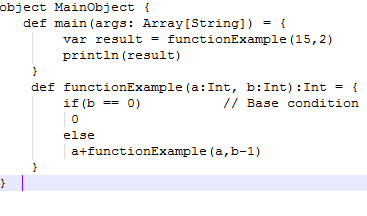Output: 30

### Scala Strings

String is an amalgamation or sequence of characters. In strings, we use a sequential approach for storing the elements in the memory. In Scala, string data structure is immutable.

String Example:

``````Class stringExample1 {

var s2 = “First String Example”

def show1(){

printf(s2)

}
}
``````

### String Methods

#### 1) Equals() method

In string, we use equals() method for comparing the two string objects. It returns true if both the string objects are equal, else it returns false.

#### 2) CompareTo() method

We use the compareTo() method for comparing the provided string with the existing string lexicographically.

#### 3) Concatenation “+” Method

We can concatenate two strings by using the “+” operator.

#### 4) Substring() method

We use a substring method for getting a substring from the given string. By mentioning the starting index and ending index as arguments, we can retrieve the substring based on our requirement.

### Scala Array

Array is a group of changeable values. From Arrays, we can retrieve the elements based on the index of the element. Index starts from 0 and ends at n-1, where “n” is the size of the array.

Scala supports two kinds of Arrays; they are:

#### 1) Single Dimensional Array

Single Dimensional Array stores the elements in sequential order. We store the array elements in the contiguous memory area.

Syntax of Single Dimensional Array is as follows:

Var arrayName : Array[arrayType] = new Array[arrayType](arraySize);

#### 2) Multi-Dimensional Arrays

We use multi-dimensional arrays for doing matrix operations and developing tables.

Example:

Var Matrix1 = 0fDim[Int] (4,4)

### Exception Handling

In Scala Programing Language, we use exception handling for handling the unusual conditions. Through exception handling, we can terminate the program unexpectedly. Scala does not have checked exceptions, it has only unchecked exceptions. Scala has certain mechanisms to handle the exceptions; they are as follows:

#### 1) Try-Catch Block

For handling the exceptions, Scala provides Try-Catch Block. Try block encloses the suspicious code. A catch block handles the exception that occurs in the Try block. In one program, we can have any number of try-catch blocks.

Example:

``````Class Try-Catch{

def division(x:Int, y:Int) = {

try {

x/y

}catch{

case z: ArithmeticException => println(z)

}

println(“Execute the rest of the program”)

}
}

object main1{

def main(args: Array[String]) {

var z = new Try-Catch()

z.division(50,0)

}
}
``````

When we execute the above code, we get the following output:

java.lang.ArithmeticException: / by zero

Execute the rest of the program.

#### 2) Finally Block

Finally, block releases the resources during the exception. The resources can be a network connection, database connection, files, etc.

#### 3) Throw Keyword

We can throw the exceptions externally in our code. Scala Programming Language uses the  “Throw” keyword for throwing the exceptions. It is mainly useful for throwing the customised exceptions.

#### 4) Throws Keyword

Scala uses throws keyword for declaring the exceptions. By using the method definition, we can declare the exception. “Throws” keyword gives information to the caller function that this method can throw the exception.

### Scala Collections

Scala has a broad range of collection libraries. It has classes and traits for collecting the data. Collections can be immutable or mutable, and we can use them based on our requirement.

The “scala.collection.mutable” package has mutable collections, and we can remove, update and add the data when we use this package. The “scala.collection.immutable” package has immutable collections. It will not allow us to change the data. By default, scala imports this package.

#### Types of Collections

1) Set

We use set collection for storing the distinct elements in a set. It will not use a particular order for storing the elements. We define the Set collection in the “scala.collection.immutable” package.

2) List

We use List collection for storing the ordered elements. It acts as a class to immutable linked lists. It is suitable for the LIFO(Last-In-First-out) approach. It stores elements to order and replicate the elements

3) Maps

Maps store the elements in pairs of values and keys. We can create a map by using a comma or rocket parameter.

4) Tuples

Unlike a list or an array, tuple stores the objects with different data types.

5) Options

Options have a container to zero or one element of the given data type.

### File Handling

Scala contains some inbuilt methods to handle the files. We can generate, open and close the file. It uses the “java.io” package for reading and writing the files. For creating the files, we use java “FileWriter” or “PrintWriter” methods.

Example:

``````Import java.io

val fileobject1 = new File(“Scala1.txt”)
val printwriter = new PrintWriter(fileObject1)

printwriter.write(“First Scala File”)

printwriter.close()
``````

The above scala code will create a file with “Scala1.txt” name.

### Scala Traits

Trait binds the method and field definitions, that can be reused by combining them with classes. One class can blend unlimited traits. We use traits for defining the objects by mentioning the signature of the supported methods. The definition of traits is similar to the class definition.

For defining the traits, we use the “trait” keyword. As child classes can implement the un-implemented methods of traits, we can say that traits are similar to the abstract classes of the java.

Example:

``````trait A{

def print1()

}

Class 	B extends A{

def print1(){

println(“Hai”)

}
}

Object Main1{

def main(args:Array[String]) {

var c = new B()

c.print()

}
}
``````

### Scala Tuples

Tuple is a collection of ordered elements. If a tuple does not have any element, then it is known as an empty tuple. In tuples, we can store any kind of data. Through Tuples, we can return multiple values from the functions.

Conclusion

Scala is an object-oriented programming language, and it has all the features of java programming. It is hugely used in Big data for handling large amounts of data. If you know java programming, then you can learn scala programming easily. I hope this Scala Tutorial article provides you with the required information about Scala Programming.

If you have any queries, let us know by commenting in the below section.Stay updated with our newsletter, packed with Tutorials, Interview Questions, How-to's, Tips & Tricks, Latest Trends & Updates, and more ➤ Straight to your inbox!

Course Schedule
NameDates
Apache Scala TrainingSep 27 to Oct 12
Apache Scala TrainingOct 01 to Oct 16
Apache Scala TrainingOct 04 to Oct 19
Apache Scala TrainingOct 08 to Oct 23
Last updated: 25 September 2022